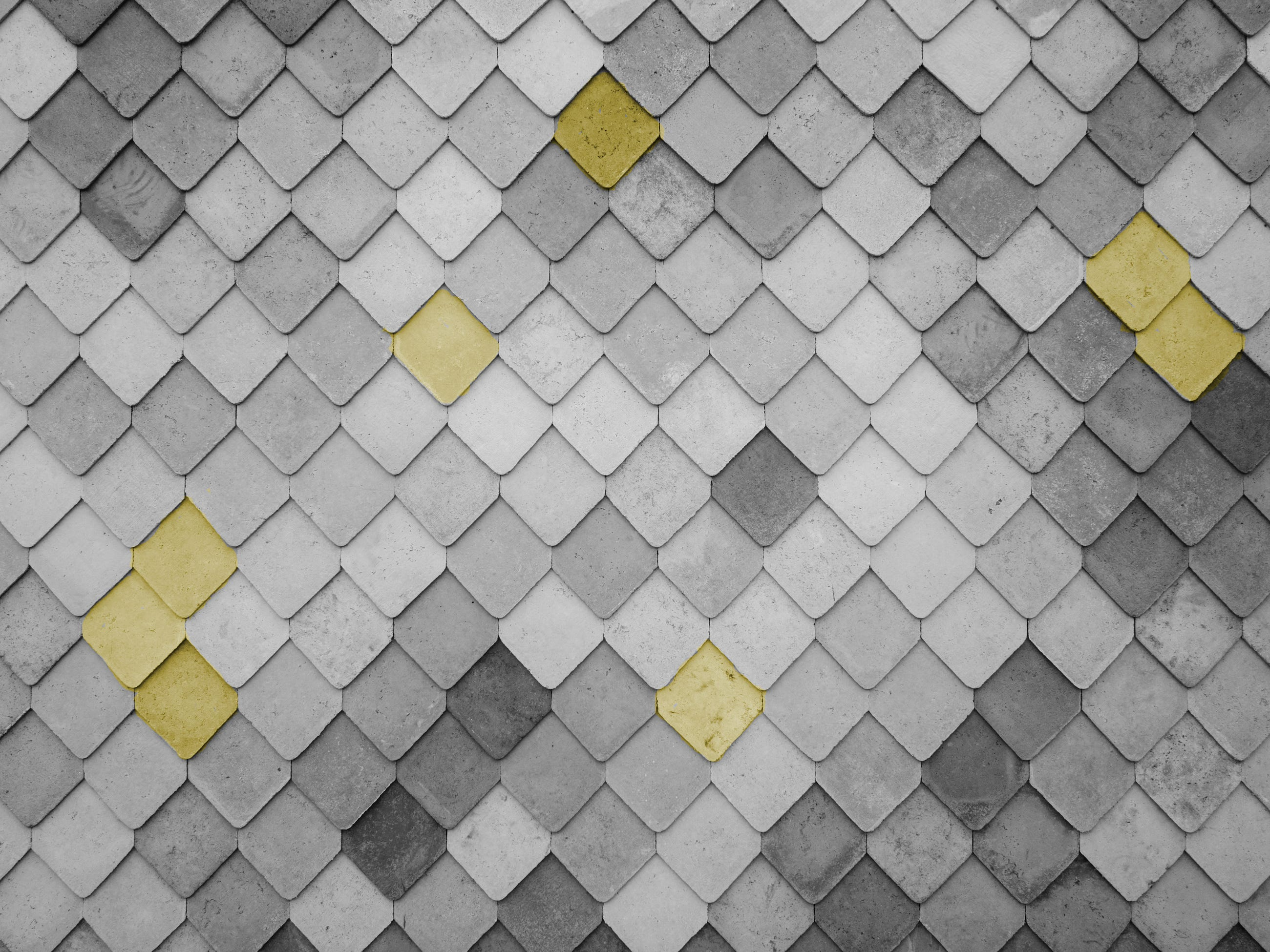# Image Augmentations with Albumentations

Original article was published by Derrick Mwiti on Deep Learning on MediumBefore we can apply the transformation, we have to convert the image to a NumPy array.

Now, let’s apply the transformation.

We can also visualize the transformed image using Matplotlib.

Since these transformations are random, the image you’ll see will definitely be different from this one.

## Color Augmentations

Let’s now try out different augmentations starting with color augmentations.

We can, for example, change the lighting using the brightness contrast.

Let’s visualize the transformed image. Compare this to the original one and you’ll notice some change in lighting.

Let’s see how we can randomly change the saturation, hue, and value of the image.

When we transform and visualize the image, we get the image below. Again, this might be different for you.

Another transformation we can do is to randomly rearrange the channels of the RGB image. Here’s the result I obtained when doing this:

Let’s look at how this rearrangement can be accomplished. First, define the transformation pipeline.

With that in place, transform and visualize the image.

The process of performing different visualizations is the same. Let me show you one more.

We can mimic snowing in our images using the `RandomSnow` transformation.

Let’s visualize it.